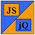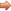# JavaScript & jQuery Tutorials

JS HomeJS ReferenceMathatan2()

## atan2()`Math`  class method

### Description

Returns a numeric radians value between -`PI` and `PI` representing the anticlockwise angle theta of an (x,y) point.

• Radians to Degrees - divide number by (`Math.PI` / 180).
• Degrees to Radians - multiply number by (`Math.PI` * 180).
• Like all class methods `atan2()` should be used on the class rather than an instance of the class.

### Syntax

Signature Description
`Math.atan2(y,x)`Returns a numeric radians value between -`PI` and `PI` representing the anticlockwise angle theta of an (x,y) point.

### Parameters

Parameter Description
`y`y-axis coordinate:
• Non-numeric strings returns `NaN`.
• Passing no parameter returns `NaN`.
• Passing `null` returns 0.
`x`x-axis coordinate:
• Non-numeric strings returns `NaN`.
• Passing no parameter returns `NaN`.

### Examples

The code below displays` atan2()` usage.

``````

// Store results in an array.
var atan2Values = new Array(10);
atan2Values = Math.atan2(-7,2);
atan2Values = Math.atan2(7,-2);
atan2Values = Math.atan2(0,5);
atan2Values = Math.atan2(5,0);
atan2Values = Math.atan2('0.75','2');
atan2Values = Math.atan2('a string',6);
atan2Values = Math.atan2(6,'a string');
atan2Values = Math.atan2();
atan2Values = Math.atan2(null,4);
atan2Values = Math.atan2(4,null);

``````

Press the button below to action the above code:

## Related Tutorials

JavaScript Advanced Tutorials - Lesson 4 - Math